# 链家广州二手房的数据与分析——数据分析1

### Inspect Data

print(subset(house, total_price == max(total_price))) #誉峰 2800w
print(subset(house, total_price == min(total_price))) #荔城中区 50w
print(subset(house, unit_price == max(unit_price))) #天銮 121433
print(subset(house, unit_price == min(unit_price))) #富力金港城南区 7471
print(mean(house$total_price)) # 268.3635w print(mean(house$unit_price)) # 34914.52
print(median(house$total_price)) # 228w print(median(house$unit_price)) # 33216


print(subset(house, follow == max(follow))) # 金影花园
print(subset(house, follow == min(follow) & release_time >= 60)) #花东镇 太平镇 凤凰城 等


### Data Visualization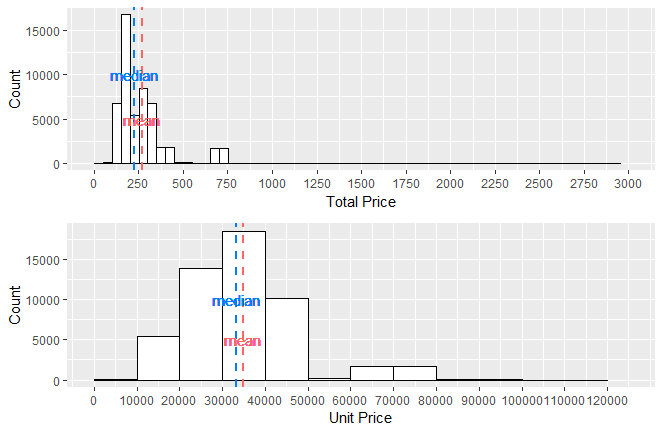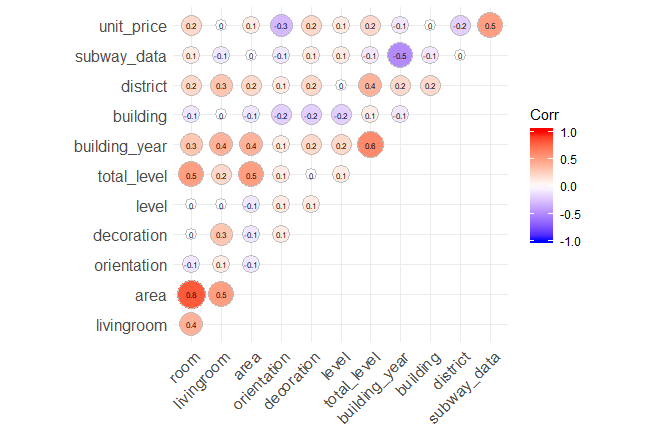##### 户型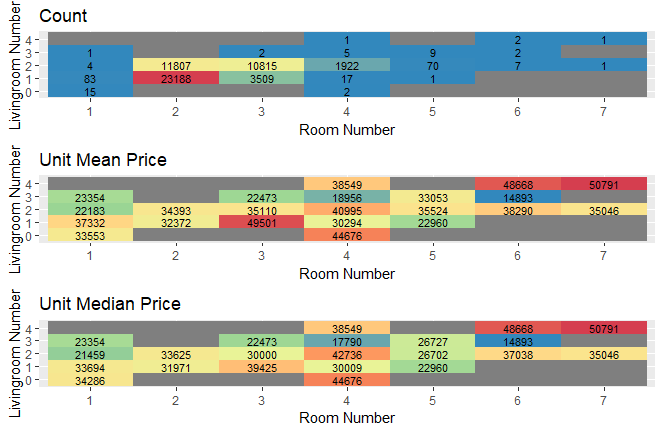##### 面积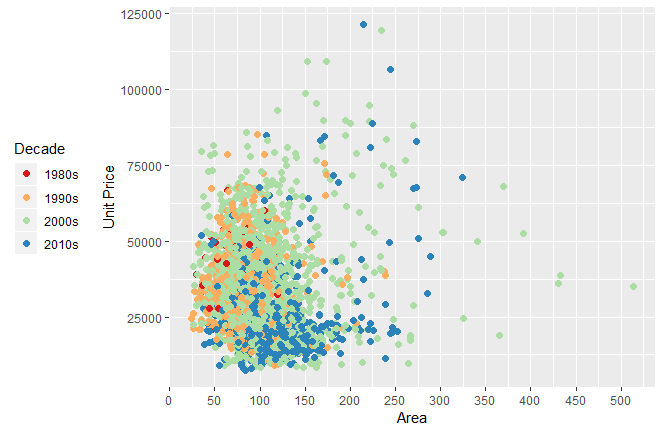##### 交通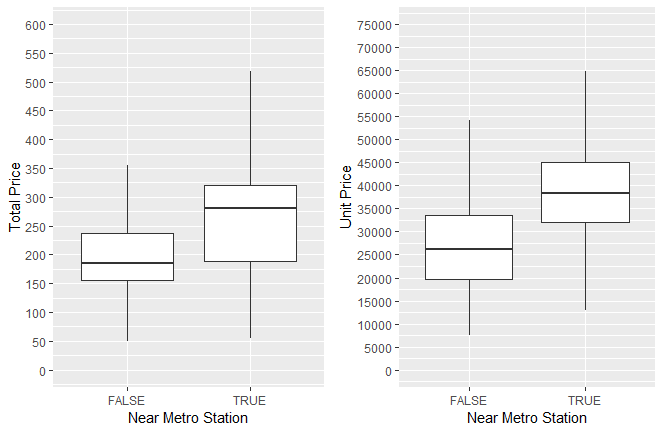##### 建筑年代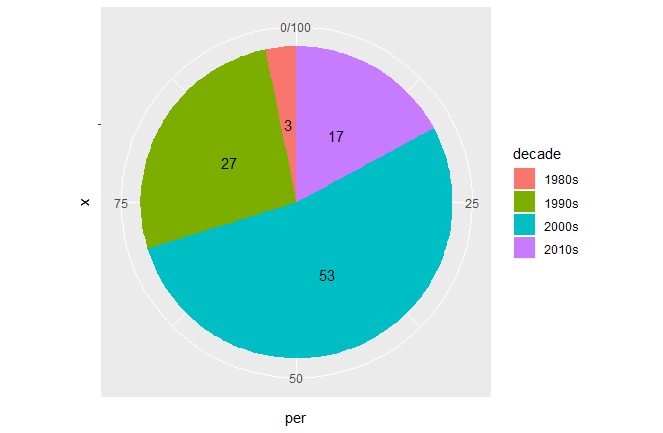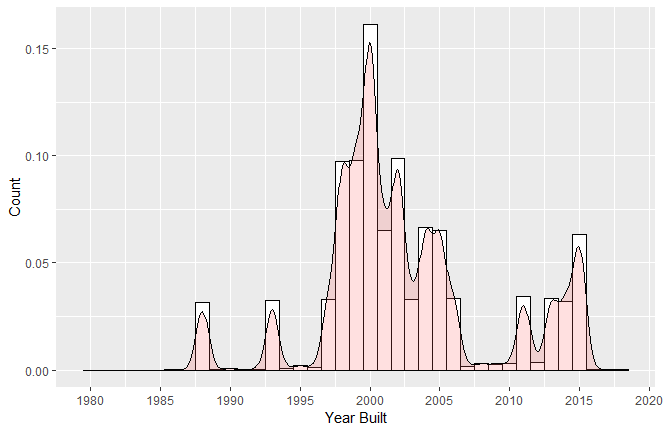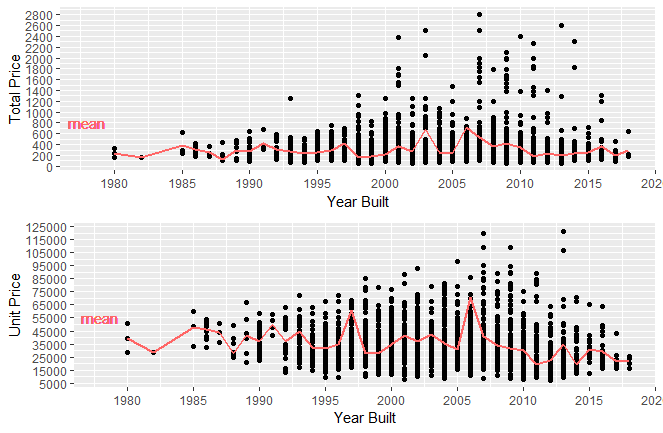##### 关注度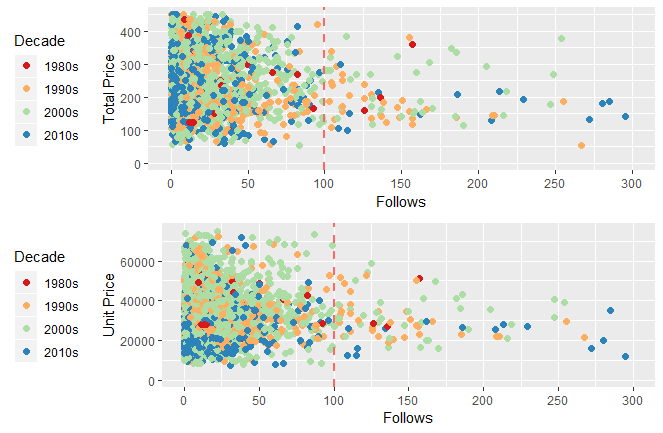##### 税费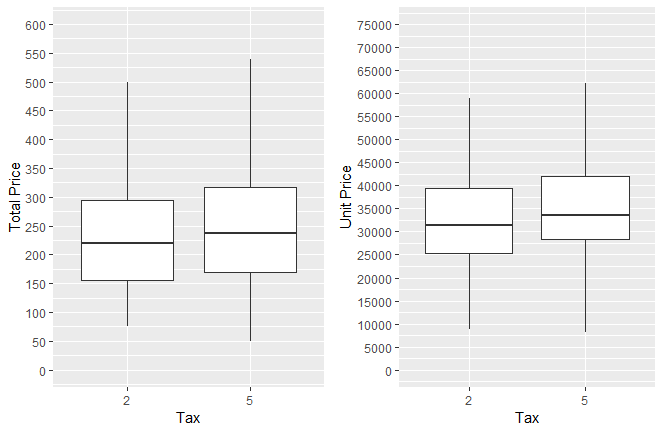##### 朝向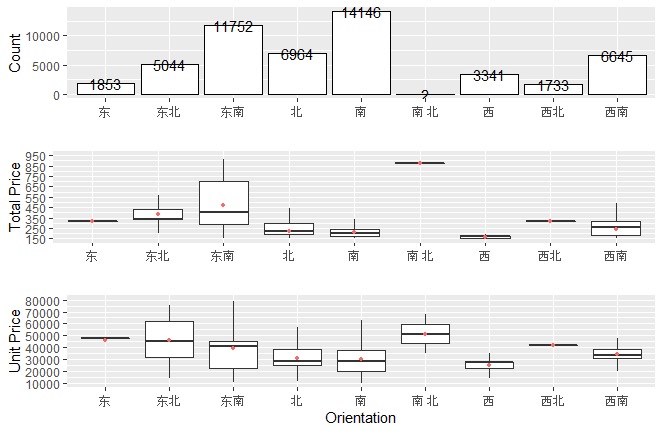##### 装修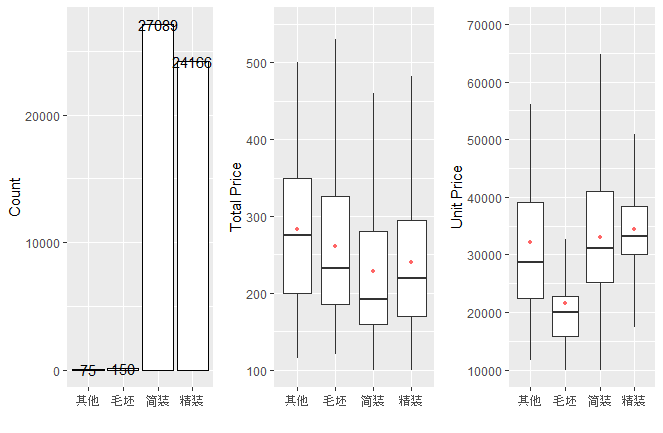##### 区域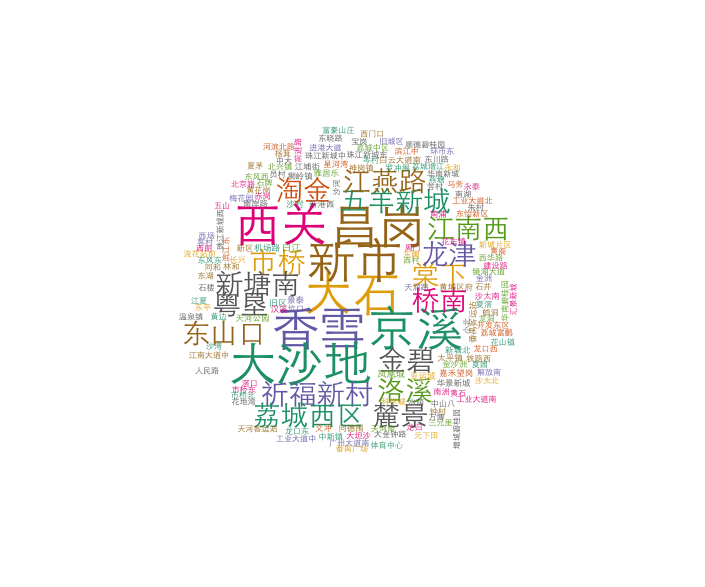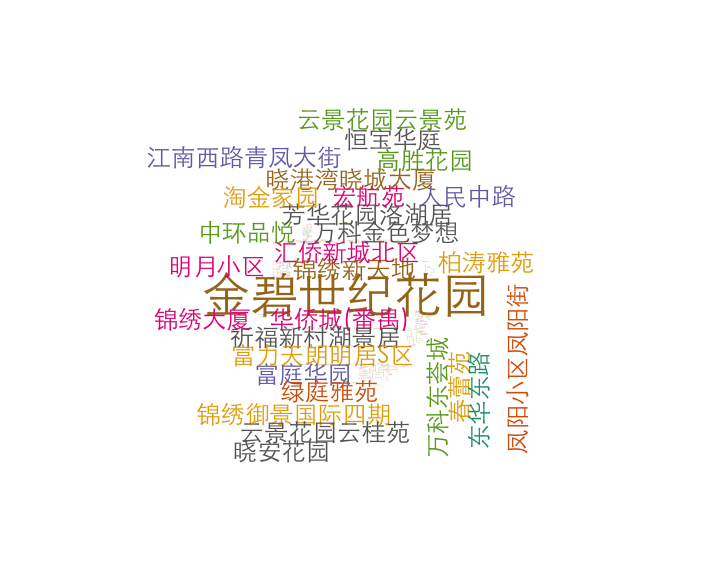##### 其他### To Be Continued

posted @ 2019-07-30 19:30  yukiwu  阅读(3806)  评论(5编辑  收藏  举报